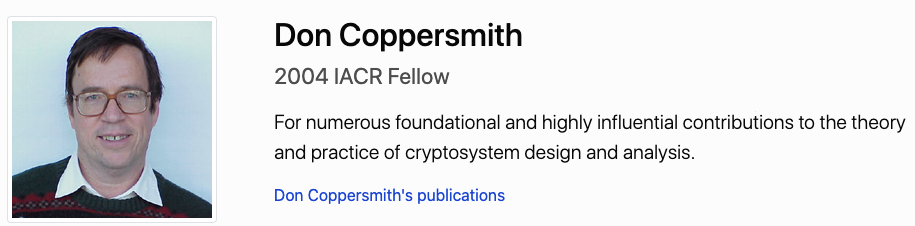# Case Study: Coppersmith Related Attack - An Academical Approach

## Introdcution

A collection of some coppersmith-related-attack academical papers (for further researching).

## Timeline

• (1996, 2001) Coppersmith introduced two methods for finding small roots of polynomial equations using lattice reduction.1. D. Coppersmith. $\textcolor{yellow}{\text{Finding a Small Root of a Bivariate Integer Equation}}$; Factoring with high bits known. In Advances in Cryptology-Eurocrypt ’96, Lecture Notes in Computer Science, volume 1070, pages 178–189. Springer-Verlag, 1996.

2. D. Coppersmith. $\textcolor{yellow}{\text{Finding a Small Root of a Univariate Modular Equation}}$. In Advances in Cryptology-Eurocrypt ’96, Lecture Notes in Computer Science, volume

1070, pages 155–165. Springer Verlag, 1996.

3. D. Coppersmith. $\textcolor{yellow}{\text{Finding Small Solutions to Small Degree Polynomials}}$. In Cryp-

tography and Lattice Conference, Lecture Notes in Computer Science, volume 2146.

Springer-Verlag, 2001.

• (1997, 2004) Howgrave-Graham & Coron revisted Coppersmith’s ideas and proposed alternative constructions1. N. Howgrave-Graham. $\textcolor{yellow}{\text{Finding Small Roots of Univariate Modular Equations Revisited}}$.

In Proceedings of the 6th IMA International Conference on Cryptography

and Coding, pages 131–142, London, UK, 1997. Springer-Verlag.

2. J.-S. Coron. $\textcolor{yellow}{\text{Finding Small Roots of Bivariate Integer Polynomial Equations Revisited}}$.

In Advances in Cryptology-Eurocrypt ’04, Lecture Notes in Computer Science,

pages 492–505. Springer-Verlag, 2004.

• (2005) Bl¨omer and May present new results using Coppersmith’s method for polynomials whose shapes are more complicated than those originally considered in Coppersmith’s articles.

1. JBlomer and A. May. A Tool Kit for $\textcolor{yellow}{\text{Finding Small Roots of Bivariate Polynomials over the}}$ $\textcolor{yellow}{\text{Integers}}$. Proceedings of Eurocrypt 2005, Lecture Notes in Computer Science, 3494:251–257, 2005.
• (2001) Howgrave-Graham explains how to cast the problem of finding roots for particular polynomials in the more general context of approximate GCD computations.

1. N. Howgrave-Graham. $\textcolor{yellow}{\text{Approximate Integer Common Divisor}}$. In CaLC ’01: Lecture Notes in Computer Science, volume 2146, pages 51–66. Springer-Verlag, 2001
• (2005) Some researchers try to adapt all these methods for more than two variables.

1. M. Ernst, E. Jochemsz, A. May, and B.de Weger. $\textcolor{yellow}{\text{Partial Key Exposure Attacks on RSA up to}}$ $\textcolor{yellow}{\text{Full Size Exponents}}$. In Advances in Cryptology (Eurocrypt 2005), Lecture Notes in Computer Science Volume 3494, pages 371-386, Springer-Verlag, 2005.
• (2001, 2004, 2005) Problem encountered with more than two variables: CANNOT guarantee that the polynomial outputted by LLL reduction are algebraically independent. Some researches about the problem:

1. J. Bl¨omer and A. May. $\textcolor{yellow}{\text{Low Secret Exponent RSA Revisited}}$. In CaLC ’01: Revised Papers from the International Conference on Cryptography and Lattices, pages 4– 19, London, UK, 2001. Springer-Verlag.
2. M. J. Hinek. $\textcolor{yellow}{\text{New partial key exposure attacks on RSA revisited}}$. Technical report, CACR, Centre for Applied Cryptographic Research, University of Waterloo, 2004.
3. M. J. Hinek. $\textcolor{yellow}{\text{Small Private Exponent Partial Key-Exposure Attacks on Multiprime RSA}}$. Technical report, CACR, Centre for Applied Cryptographic Research, University of Waterloo, 2005.
• (2007) Aur´elie Bauer and Antoine Joux propose a new generalization of Coppersmith’s method in three variables, using a new lattice construction to find a third independent polynomial. Their construction uses Gr¨obner basis in addition to lattice reduction.

1. Bauer, Aurélie, and Antoine Joux. “$\textcolor{yellow}{\text{Toward a rigorous variation of Coppersmith’s algorithm}}$ $\textcolor{yellow}{\text{on three variables}}$.” Annual International Conference on the Theory and Applications of Cryptographic Techniques. Springer, Berlin, Heidelberg, 2007.
• Real-world attack using coppersmith method.

1. (2013) Bernstein, Daniel J., et al. “$\textcolor{yellow}{\text{Factoring RSA keys from certified smart cards:}}$ $\textcolor{yellow}{\text{Coppersmith in the wild}}$.” International Conference on the Theory and Application of Cryptology and Information Security. Springer, Berlin, Heidelberg, 2013.

2. (2017) Nemec, Matus, et al. “$\textcolor{yellow}{\text{The return of coppersmith’s attack: Practical factorization of}}$ $\textcolor{yellow}{\text{widely used rsa moduli}}$.” Proceedings of the 2017 ACM SIGSAC Conference on Computer and Communications Security. 2017. $$p = k \cdot M + ({65537}^a \bmod{M})$$

• (2010) A nice overview on various attacks on RSA based on Coppermith’s algorithm

1. May, Alexander. “$\textcolor{yellow}{\text{Using LLL-reduction for solving RSA and factorization problems}}$.” The LLL algorithm. Springer, Berlin, Heidelberg, 2009. 315-348.